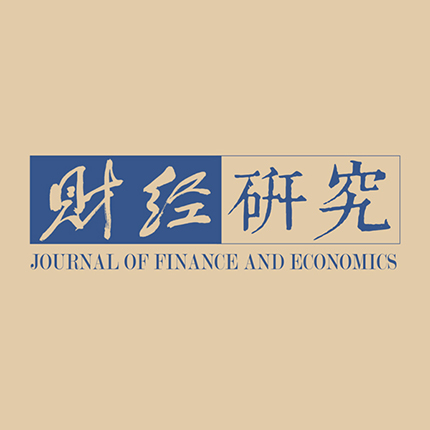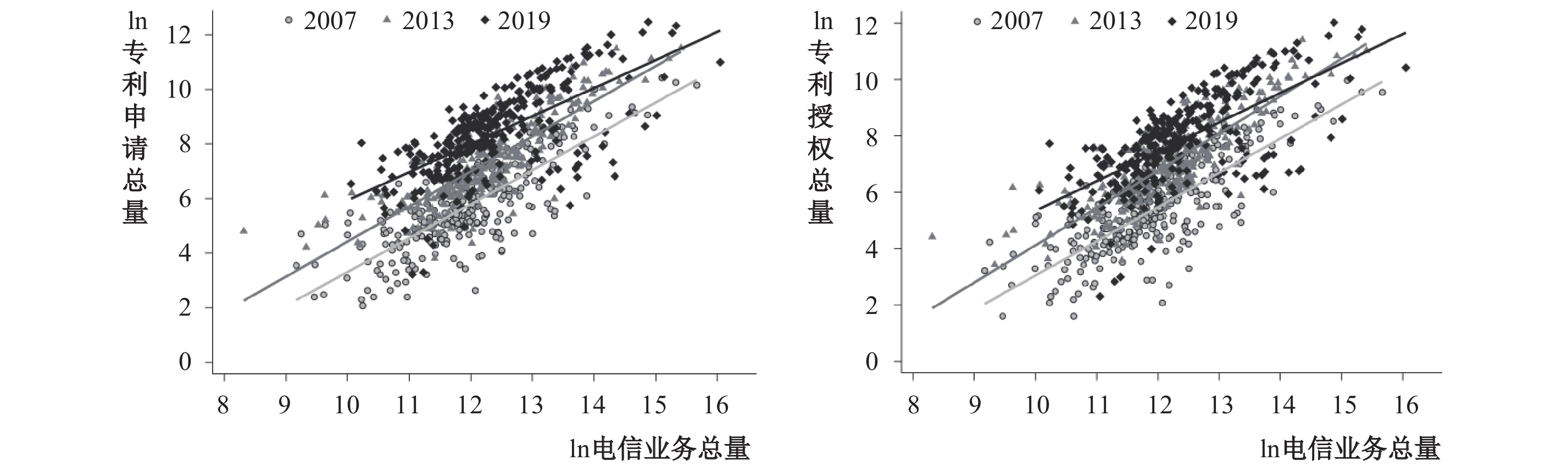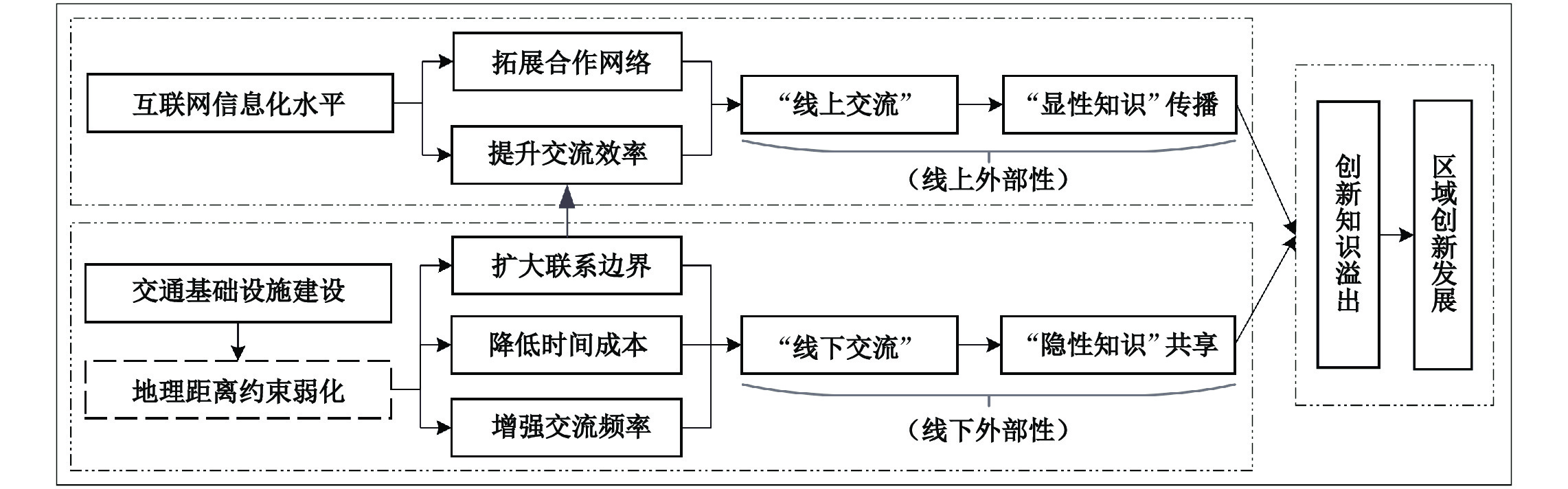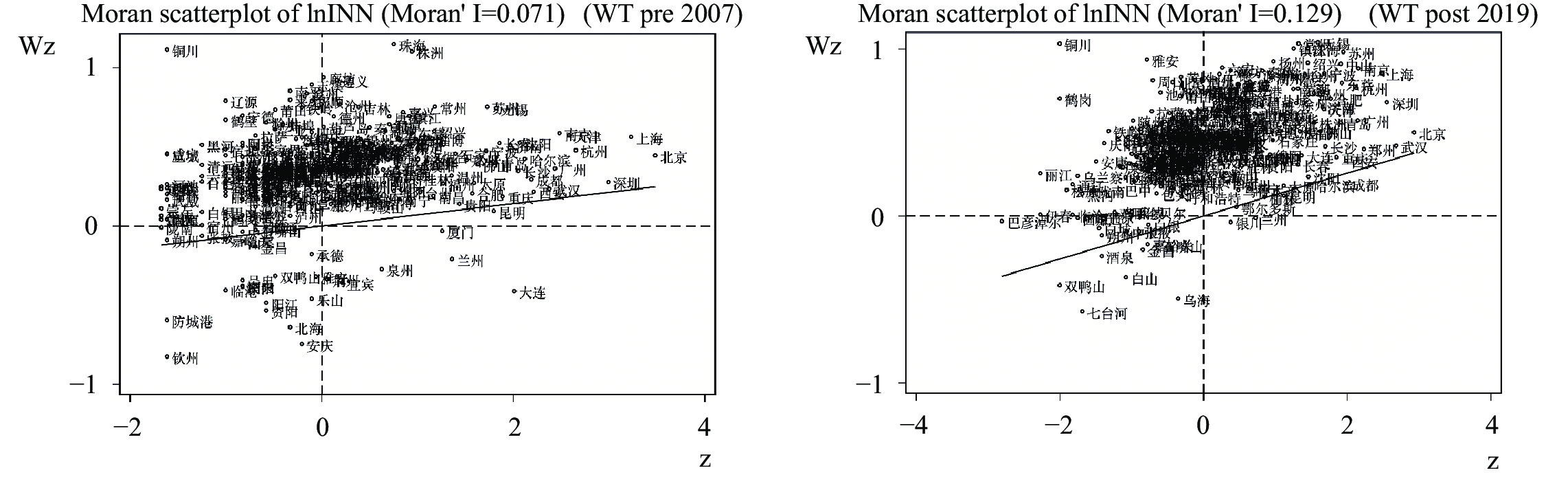﻿ 互联网时代“线下交流”不再重要了吗？《财经研究》
2023第49卷第3期

1. 上海交通大学 安泰经济与管理学院, 上海 200030;
2. 南昌大学 经济管理学院, 江西 南昌 330031;
3. 重庆大学 经济与工商管理学院, 重庆 400044

Is “Offline Communication” No Longer Important in the Internet Era? Test from the Paradox of “Knowledge Spatial Spillover” and Regional Innovation
Zheng Weiwei1, Qu Xi1, Liu Yaobin2, An Linglin3
1. Antai College of Economics and Management, Shanghai Jiao Tong University, Shanghai 200030, China;
2. School of Economics and Management, Nanchang University, Nanchang 330031, China;
3. School of Economics and Business Administration, Chongqing University, Chongqing 400044, China
Summary: Innovation is the primary driving force behind development. In the Internet era, innovation knowledge spillover is faced with two paradoxes: One is the increasing frequency of “online communication” and the decreasing opportunity of “offline communication”, and the other is the insufficient innovation effect of “codified knowledge” and the limited communication channel of “tacit knowledge”. By using the level of the Internet informatization and the construction of transportation infrastructure to measure the intensity of “online communication” and “offline communication” respectively, and collecting a sample of 283 prefecture-level cities from 2007 to 2019, this paper applies the spatial panel Durbin model to study the impact of online “codified knowledge” communication and offline “tacit knowledge” sharing on the quantity and quality of regional innovation. The results indicate that, after controlling the spillover effect of knowledge, “online communication” and “offline communication” cannot completely substitute each other. Although “online communication” can effectively increase the innovation quantity in local and adjacent areas, it has a limited effect on innovation quality. Only by combining the efficiency of “online communication” represented by the Internet informatization with the improvement of “offline communication” represented by high-speed rail construction, one can make the comprehensive utilization of “codified knowledge” and “tacit knowledge” to improve the quality of regional innovation. The main contributions of this paper are as follows: First, it distinguishes the different transmission channels between “codified knowledge” and “tacit knowledge”, the former mainly through “online communication” and the latter through “offline communication”, and points out that the combination of “online communication” and “offline communication” is the key to solve the paradox of “knowledge spillover”. Second, it acquires data of transportation time and frequency between cities to capture the improvement of “offline communication” by weakening the geographical distance. Third, it applies the two-way fixed effect spatial panel Durbin model to analyze the spatial spillover from neighboring areas, and systematically investigate the effect of the Internet and transportation infrastructure on the quantity and quality of regional innovation.
Key words: online communication    offline communication    codified knowledge    tacit knowledge    regional innovation development图 1 2007年、2013年和2019年中国信息化水平与区域创新水平散点图

（一）知识空间溢出与区域创新发展

（二）互联网信息化水平、“显性知识”与区域创新发展图 2 互联网发展和交通基础设施建设对创新的影响机理

（三）交通基础设施建设、“隐性知识”与区域创新发展

（一）空间面板杜宾模型

 $\begin{array}{c}{Y}_{it}=\rho {\sum }_{j=1}^{n}{\mathit{W}}_{ij}{Y}_{jt}+{\sum }_{j=1}^{n}{\mathit{W}}_{ij}{\mathrm{l}\mathrm{n}INT}_{jt}\theta +{\sum }_{j=1}^{n}{\mathit{W}}_{ij}{\mathit{X}}_{jt}\widetilde{\theta }+{\mathrm{l}\mathrm{n}INT}_{it}\beta +{\mathit{X}}_{it}\widetilde{\beta }+{\alpha }_{i}+{\gamma }_{t}+{\varepsilon }_{it}\end{array}$ (1)

（二）变量说明

1. 被解释变量：区域创新水平（lnPAT和lnINN）。已有文献大多采用专利申请和授权量、新产品市场价值、专利引用量与城市创新指数等衡量区域创新水平。考虑到发展中国家创新能力仍处于模仿和学习过程中，专利是一个更能体现创新活动价值的指标（宛群超和袁凌，2021）。相较于专利申请量侧重从投入角度体现创新活动，专利授权量能够更好地体现创新的实际产出数量（陈大峰等，2020）。此外，发明专利因其申请难度最大、技术含量最高等特性，可以更好地反映创新成果质量（余泳泽等，2021）。因此，本文分别选择专利总授权和发明专利授权量衡量区域创新成果数量和质量。

2. 核心解释变量：互联网信息化水平（lnINT）。本文采用电信业务总量作为互联网信息化水平的代理变量。“国际互联网用户数”单纯用数量刻画地区互联网信息化水平，而“电信业务总量”通过价格衡量电信企业为社会提供的各类电信服务的总数量，这能够更加真实地反映地区实际建设和应用的互联网信息化水平。

3. 控制变量。为避免其他潜在因素对区域创新水平的影响，本文控制了科研财政投入力度（lnRD）、地区经济发展水平（lnPGDP）、人力资本投资（lnSTU）、市场规模（lnMarket）、环境规制强度（lnSO2）、生产性服务业专业化（lnMAR）和多样化集聚程度（lnJacobs），公式为：

 ${MAR}_{it}= \sum _{j=1}^{n}\;\left|\dfrac{{E}_{ijt}}{\sum _{j=1}^{n}\;{E}_{ijt}}-\dfrac{\sum _{i=1}^{m}\;{E}_{ijt}}{\sum _{i=1}^{m}\;\sum _{j=1}^{n}\;{E}_{ijt}}\right|$ (2)
 ${Jacobs}_{it}=\dfrac{1}{\sum _{j=1}^{n} {\left[\dfrac{{E}_{ijt}}{\sum _{j=1}^{n} {E}_{ijt}-{E}_{ijt}}\right]}^{2}}/\dfrac{1}{\sum _{j=1}^{n} {\left[\dfrac{\sum _{i=1}^{m}{E}_{ijt}}{\sum _{i=1}^{m} \sum _{j=1}^{n} {E}_{ijt}-\sum _{i=1}^{m}{E}_{ijt}}\right]}^{2}}$ (3)

4. 空间权重矩阵。由于传统对称空间权重矩阵既无法区分发达与不发达地区知识空间溢出效应的差异，又不具备时变特征，无法体现近年来交通基础设施建设对研发人员“线下交流”的影响，本文采用了地区间每日列车实际通行时间和发车频次数据。Yu和Fan（2018）采用地区间地理距离除以各类型列车设计时速来衡量通行时间，而本文方法具有两大优势：第一，能够避免忽视不同地区交通线路实际运行时速存在的差异，更好地反映实际情况；第二，可以最大程度地缓解构建的空间权重矩阵与现实的偏差，确保参数估计结果可靠。综上所述，本文区分了高铁开通前（pre）、后（post）两个阶段，分别构建了283个地级市间双向非对称的反通行时间距离（ ${W}_{T}$ ）和发车频次（ ${W}_{F}$ ）空间权重矩阵：

 {W}_{{T}_{ij}}= \left\{ \begin{aligned}&1 / min \{{T}_{ij}\}\quad {{if}}\;i \ne j \\ &0 \quad\quad\quad\quad\;\;\;{{if}}\;i=j \end{aligned} \right. (4)
 {W}_{{F}_{ij}}=\left\{\begin{aligned}& \sum {F}_{ij}\quad\quad{{if}}\;i\ne j\\& 0\quad\quad\quad\quad{{if}}\;i=j\end{aligned}\right. (5)

（三）数据说明

 变量名 变量含义 样本量 标准差 最小值 均值 最大值 lnPAT 专利总授权量取对数 3679 1.7537 1.6094 6.8003 12.0234 lnINN 发明专利授权量取对数 3679 1.9631 0.0000 4.3813 10.8804 lnINT 互联网信息化水平取对数 3679 1.0149 8.3121 12.1955 16.2120 lnMAR 产业专业化集聚取对数 3679 0.4023 −2.7348 −0.9902 0.4820 lnJacobs 产业多样化集聚取对数 3679 0.7772 −6.2552 −0.8619 0.9516 lnRD 科研财政支出取对数 3679 1.4246 −1.0800 9.8546 15.2526 lnPGDP 人均GDP取对数 3679 0.6403 4.5449 10.3130 12.8692 lnSTU 每万人大学生数取对数 3679 1.2214 −2.3656 4.5338 7.1787 lnMarket 社会消费品零售总额取对数 3679 1.0889 5.2323 15.1341 18.5759 lnSO2 工业二氧化硫排放量取对数 3679 1.2350 0.6931 10.1990 13.4341

（一）空间自相关检验图 3 区域创新成果质量的全局莫兰指数散点图

（二）基准回归结果分析

 lnPAT lnINN （1） （2） （3） （4） （5） （6） （7） （8） ${W}_{T\left(pre\right)}$ ${W}_{T\left(p\mathrm{o}\mathrm{s}\mathrm{t}\right)}$ ${W}_{F\left(pre\right)}$ ${W}_{F\left(p\mathrm{o}\mathrm{s}\mathrm{t}\right)}$ ${W}_{T\left(pre\right)}$ ${W}_{T\left(p\mathrm{o}\mathrm{s}\mathrm{t}\right)}$ ${W}_{F\left(pre\right)}$ ${W}_{F\left(p\mathrm{o}\mathrm{s}\mathrm{t}\right)}$ $\rho$ 0.8574*** 0.8504*** 0.5433*** 0.6554*** 0.6896*** 0.6984*** 0.3946*** 0.4939*** （0.0342） （0.0309） （0.0609） （0.0553） （0.0553） （0.0628） （0.0459） （0.0687） lnINT 0.0448** 0.0352* 0.0491** 0.0336* 0.0162 −0.0003 0.0184 −0.0030 （0.0196） （0.0197） （0.0199） （0.0200） （0.0270） （0.0269） （0.0271） （0.0269） W×lnINT 0.3646*** 0.5009*** 0.1927*** 0.3291*** 0.0526 0.4019** 0.0158 0.2729** （0.1057） （0.1414） （0.0581） （0.0908） （0.1231） （0.1761） （0.0675） （0.1130） 控制变量 控制 控制 控制 控制 控制 控制 控制 控制 W×控制变量 控制 控制 控制 控制 控制 控制 控制 控制 个体效应 控制 控制 控制 控制 控制 控制 控制 控制 时间效应 控制 控制 控制 控制 控制 控制 控制 控制 N 3679 3679 3679 3679 3679 3679 3679 3679 R2 0.448 0.402 0.732 0.658 0.573 0.536 0.760 0.679 注：括号内为标准误；***、**和*分别表示在1%、5%和10%显著性水平下显著，下表同。

（一）采用外生的反地理距离空间权重矩阵进行内生性探讨

 {W}_{D}=\left\{\begin{aligned} &1/{d}_{ij}^{2}\quad\quad{{if}}\;i\ne j\\ & 0\quad\quad\quad\;{{{if}}}\;i=j\end{aligned}\right. (6)

 （1） （2） （3） （4） （5） （6） （7） （8） ${W}_{D}$ ${W}_{D}$ ${W}_{F\left(pre\right)}$ ${W}_{F\left(p\mathrm{o}\mathrm{s}\mathrm{t}\right)}$ ${W}_{N\left(pre\right)}$ ${W}_{N\left(p\mathrm{o}\mathrm{s}\mathrm{t}\right)}$ ${W}_{N\left(pre\right)}$ ${W}_{N\left(p\mathrm{o}\mathrm{s}\mathrm{t}\right)}$ lnPAT lnINN lnINN lnINN lnPAT lnPAT lnAPP lnAPP 直接效应 0.0728*** 0.0239 0.0406 0.0158 0.1267*** 0.1259*** 0.1358*** 0.1330*** （0.0242） （0.0284） （0.0490） （0.0189） （0.0396） （0.0370） （0.0426） （0.0410） 间接效应 7.5449*** 1.6285 −0.3037 0.1125*** 0.7434** 0.8870* 0.8005** 1.3191** （2.4340） （1.5964） （4.4135） （0.0202） （0.3339） （0.5026） （0.3470） （0.6322） 总效应 7.6177*** 1.6524 −0.2631 0.1284*** 0.8701** 1.0128** 0.9363*** 1.4521** （2.4474） （1.6006） （4.4249） （0.0148） （0.3419） （0.5129） （0.3527） （0.6437）

（二）采用空间动态面板杜宾模型缓解内生性问题

 $\begin{array}{c}{Y}_{it}=\lambda {Y}_{it-1}+\eta {\sum }_{j=1}^{n}{\mathit{W}}_{ij}{Y}_{jt-1}+\rho {\sum }_{j=1}^{n}{\mathit{W}}_{ij}{Y}_{jt}+{\sum }_{j=1}^{n}{\mathit{W}}_{ij}{\mathrm{l}\mathrm{n}INT}_{jt}\theta \\ +{\sum }_{j=1}^{n}{\mathit{W}}_{ij}{\mathit{X}}_{jt}\widetilde{\theta }+{\mathrm{l}\mathrm{n}INT}_{it}\beta +{\mathit{X}}_{it}\widetilde{\beta }+{\alpha }_{i}+{\gamma }_{t}+{\varepsilon }_{it}\end{array}$ (7)

（三）替换加权嵌套空间权重矩阵进行稳健性检验

 $\begin{array}{c}{{W}_{N}}_{ij}=\dfrac{1}{2}{W}_{{T}_{ij}}^{z}+\dfrac{1}{2}{W}_{{F}_{ij}}^{z}\end{array}$ (8)

（一）互联网信息化水平、“显性知识”存量与创新

 $\begin{array}{c}\mathrm{l}\mathrm{n}Boo{k}_{it}={\beta }_{0}+{\beta }_{1}{\mathrm{l}\mathrm{n}INT}_{it}+{\beta }_{k}{\mathit{X}}_{jt}+{\alpha }_{i}+{\gamma }_{t}+{\varepsilon }_{it}\end{array}$ (9)
 \begin{aligned}{Y}_{it}=&\rho {\sum }_{j=1}^{n}{\mathit{W}}_{ij}{Y}_{jt}+{\sum }_{j=1}^{n}{\mathit{W}}_{ij}{\mathrm{l}\mathrm{n}Book}_{jt}\delta + {\sum }_{j=1}^{n}{\mathit{W}}_{ij}{\mathrm{l}\mathrm{n}INT}_{it}\theta +{\sum }_{j=1}^{n}{\mathit{W}}_{ij}{\mathit{X}}_{jt}\widetilde{\theta }\\ &+{\mathrm{l}\mathrm{n}Book}_{jt}\widetilde{\delta }+{\mathrm{l}\mathrm{n}INT}_{it}\beta +{\mathit{X}}_{it}\widetilde{\beta }+{\alpha }_{i}+{\gamma }_{t}+{\varepsilon }_{it}\end{aligned} (10)

 （1） （2） （3） ${W}_{F\left(p\mathrm{o}\mathrm{s}\mathrm{t}\right)}$ FE（Y=lnBook） ${W}_{F\left(p\mathrm{o}\mathrm{s}\mathrm{t}\right)}$ $\rho$ 0.4939*** （0.0687） 0.4414*** （0.0671） lnINT −0.0030 （0.0269） 0.0217* （0.0121） −0.0003 （0.0265） lnBook 0.1889*** （0.0375） W×lnINT 0.2729** （0.1130） 0.2833** （0.1145） W×lnBook 0.3177** （0.1328） 控制变量 控制 控制 控制 个体效应 控制 控制 控制 时间效应 控制 控制 控制 N 3679 3679 3679 R2 0.679 0.417 0.696

（二）交通基础设施建设与线下“隐性知识”交流传播

 人口流动比例 2000—2010年 2000—2020年 2005—2015年 高铁节约时间 0.0703* [0.0665] 0.1167* [0.0887] 0.0453** [0.0317] 常数项 0.0310*** [0.0000] 0.0294*** [0.0000] 0.0318*** [0.0000] 注：方括号内为p值；***、**和*分别表示在1%、5%和10%显著性水平下显著。

 东部地区 中部地区 西部地区 东北地区 （1） （2） （3） （4） （5） （6） （7） （8） ${W}_{N\left(pre\right)}$ ${W}_{N\left(p\mathrm{o}\mathrm{s}\mathrm{t}\right)}$ ${W}_{N\left(pre\right)}$ ${W}_{N\left(p\mathrm{o}\mathrm{s}\mathrm{t}\right)}$ ${W}_{N\left(pre\right)}$ ${W}_{N\left(p\mathrm{o}\mathrm{s}\mathrm{t}\right)}$ ${W}_{N\left(pre\right)}$ ${W}_{N\left(p\mathrm{o}\mathrm{s}\mathrm{t}\right)}$ 直接效应 0.0483 0.0248 −0.0325 −0.0332 0.0805 0.0347 0.0934 0.0738 （0.0567） （0.0558） （0.0544） （0.0558） （0.0492） （0.0450） （0.0731） （0.0792） 间接效应 0.0551 0.9562** −0.1393 −0.8217 0.2250 1.6564** −0.3291 −0.6997*** （0.3324） （0.4492） （0.2821） （0.6898） （0.3753） （0.7181） （0.2494） （0.1639） 总效应 0.1033 0.9811** −0.1719 −0.8550 0.3055 1.6911** −0.2357 −0.6259*** （0.3427） （0.4428） （0.2998） （0.7117） （0.3871） （0.7233） （0.2675） （0.1806）

① 以高铁为代表的交通基础设施更能够满足生产性服务业对人力资本、信息和知识快速流动的要求。本文选取的生产性服务业包括：交通运输、仓储和邮政业、信息传输、计算机服务和软件业、批发和零售业、金融业、租赁和商业服务业、科学研究、技术服务和地质勘查业以及教育业。

② 限于篇幅，检验结果省略，读者若是感兴趣可向作者索取。

③ 限于篇幅，控制变量的回归结果省略，读者若是感兴趣可向作者索取。

④ 数据来源于历年《中国城市统计年鉴》，由于本地区图书馆藏书量不受邻近地区影响，故只需采用面板固定效应回归。

⑤ 数据来源于2000年、2010年和2020年《中国人口普查年鉴》以及2005年和2015年《全国1%人口抽样调查数据》，由于历次全国人口普查和抽样调查仅公布省级层面人员跨区域流动数据，故采用各省会城市间高铁与火车通行时间差值进行匹配。

  陈大峰, 闫周府, 王文鹏. 城市人口规模、产业集聚模式与城市创新——来自271个地级及以上城市的经验证据[J]. 中国人口科学, 2020(5): 27–40.  邓涛涛, 王丹丹. 中国高速铁路建设加剧了“城市蔓延”吗?——来自地级城市的经验证据[J]. 财经研究, 2018(10): 125–137.  董艳梅, 朱英明. 高铁建设能否重塑中国的经济空间布局——基于就业、工资和经济增长的区域异质性视角[J]. 中国工业经济, 2016(10): 92–108. DOI:10.19581/j.cnki.ciejournal.2016.10.007  范欣, 宋冬林, 赵新宇. 基础设施建设打破了国内市场分割吗?[J]. 经济研究, 2017(2): 20–34.  何凌云, 陶东杰. 高铁开通对知识溢出与城市创新水平的影响测度[J]. 数量经济技术经济研究, 2020(2): 125–142. DOI:10.13653/j.cnki.jqte.2020.02.007  黄群慧, 余泳泽, 张松林. 互联网发展与制造业生产率提升: 内在机制与中国经验[J]. 中国工业经济, 2019(8): 5–23.  蒋殿春, 于洋, 方森辉. 专利试点政策如何影响城市创新水平[J]. 南开经济研究, 2021(6): 34–52.  金培振, 殷德生, 金桩. 城市异质性、制度供给与创新质量[J]. 世界经济, 2019(11): 99–123.  李建成, 陈建隆, 邓敏. 地理约束、合作与劳动力知识分配空间偏好[J]. 统计研究, 2021(11): 115–129.  刘芳. 高速铁路、知识溢出与城市创新发展——来自278个城市的证据[J]. 财贸研究, 2019(4): 14–29.  刘耀彬, 郑维伟. 不同等级高速铁路对区域经济差异的影响——基于DID模型对江西省的实证分析[J]. 南通大学学报(社会科学版), 2019(6): 23–33.  彭向, 蒋传海. 产业集聚、知识溢出与地区创新——基于中国工业行业的实证检验[J]. 经济学(季刊), 2011(3): 913–934. DOI:10.13821/j.cnki.ceq.2011.03.013  孙建军, 王树祥, 苏志文, 等. 双元创新价值链模型构建: 基于扎根理论的企业创新模式研究[J]. 管理评论, 2022(5): 340–352.  田相辉, 徐小靓. 创新生产的空间效应与结构效应[J]. 管理评论, 2018(3): 95–101. DOI:10.14120/j.cnki.cn11-5057/f.2018.03.009  宛群超, 袁凌. 创新要素流动与高技术产业创新能力[J]. 科研管理, 2021(12): 80–87. DOI:10.19571/j.cnki.1000-2995.2021.12.010  王雨飞, 倪鹏飞, 赵佳涵, 等. 交通距离、通勤频率与企业创新——高铁开通后与中心城市空间关联视角[J]. 财贸经济, 2021(12): 150–165. DOI:10.3969/j.issn.1002-8102.2021.12.011  徐德英, 韩伯棠. 地理、信息化与交通便利邻近与省际知识溢出[J]. 科学学研究, 2015(10): 1555–1563. DOI:10.3969/j.issn.1003-2053.2015.10.015  叶德珠, 潘爽, 武文杰, 等. 距离、可达性与创新——高铁开通影响城市创新的最优作用半径研究[J]. 财贸经济, 2020(2): 146–160. DOI:10.3969/j.issn.1002-8102.2020.02.010  余泳泽, 刘凤娟, 庄海涛. 互联网发展与技术创新: 专利生产、更新与引用视角[J]. 科研管理, 2021(6): 41–48.  余泳泽, 庄海涛, 刘大勇, 等. 高铁开通是否加速了技术创新外溢?—来自中国230个地级市的证据[J]. 财经研究, 2019(11): 20–31. DOI:10.16538/j.cnki.jfe.2019.11.002  张学良. 中国交通基础设施促进了区域经济增长吗——兼论交通基础设施的空间溢出效应[J]. 中国社会科学, 2012(3): 60–77.  种照辉, 高志红, 覃成林. 网络基础设施建设与城市间合作创新——“宽带中国”试点及其推广的证据[J]. 财经研究, 2022(3): 79–93.  Bloom N, Garicano L, Sadun R, et al. The distinct effects of information technology and communication technology on firm organization[J]. Management Science, 2014, 60(12): 2859–2885. DOI:10.1287/mnsc.2014.2013  Dong X F, Zheng S Q, Kahn M E. The role of transportation speed in facilitating high skilled teamwork across cities[J]. Journal of Urban Economics, 2020, 115: 103212. DOI:10.1016/j.jue.2019.103212  Huang Z K, Li L X, Ma G R, et al. Hayek, local information, and commanding heights: Decentralizing state-owned enterprises in China[J]. American Economic Review, 2017, 107(8): 2455–2478. DOI:10.1257/aer.20150592  Nonaka I, Von Krogh G. Perspective-Tacit knowledge and knowledge conversion: Controversy and advancement in organizational knowledge creation theory[J]. Organization Science, 2009, 20(3): 635–652. DOI:10.1287/orsc.1080.0412  Poleacovschi C, Javernick-Will A, Tong T, et al. Engineers seeking knowledge: Effect of control systems on accessibility of tacit and codified knowledge[J]. Journal of Construction Engineering and Management, 2019, 145(2): 04018128. DOI:10.1061/(ASCE)CO.1943-7862.0001594  Storper M, Venables A J. Buzz: Face-to-face contact and the urban economy[J]. Journal of Economic Geography, 2004, 4(4): 351–370. DOI:10.1093/jnlecg/lbh027  Van Den Berg H A. Three shapes of organisational knowledge[J]. Journal of Knowledge Management, 2013, 17(2): 159–174. DOI:10.1108/13673271311315141  Yu M, Fan W. Accessibility impact of future high speed rail corridor on the piedmont Atlantic megaregion[J]. Journal of Transport Geography, 2018, 73: 1–12. DOI:10.1016/j.jtrangeo.2018.09.014  Zhang X Z, Wu W X, Zhou Z X, et al. Geographic proximity, information flows and corporate innovation: Evidence from the high-speed rail construction in China[J]. Pacific-Basin Finance Journal, 2020, 61: 101342. DOI:10.1016/j.pacfin.2020.101342